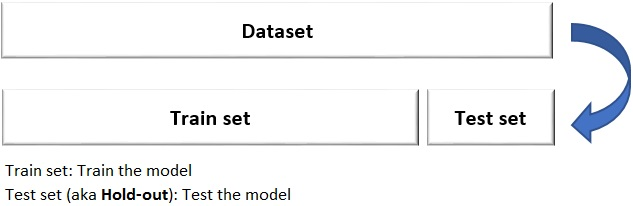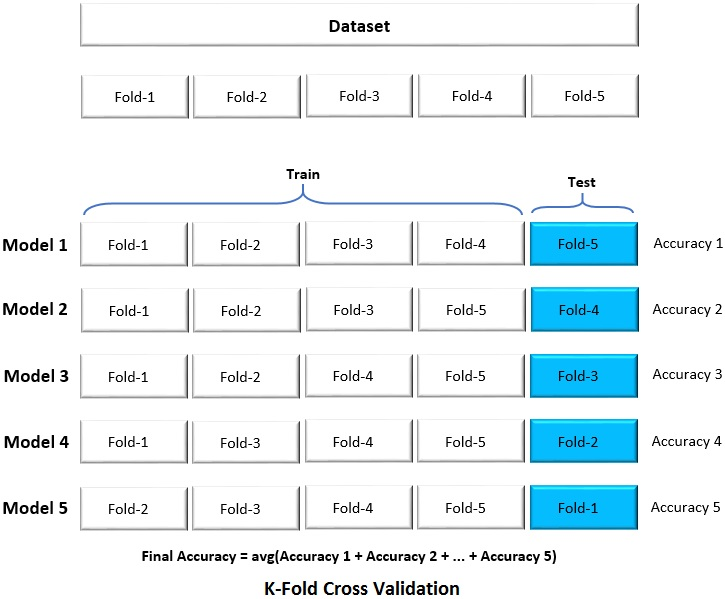# The three important Cross-Validation techniques

Cross-Validation (CV) is a model evaluation technique to compute the performance of a Machine Learning (ML) model. After building a model, validating it on trained data fetches the residual errors, and one should never use the same data to measure the quality of a model. It is required to validate it against the unseen data to arrive at the actual performance. Following are the three important CV techniques that are widely used in the industry to measure the performance:

1. Hold-out
2. K-Fold
3. Leave-One-Out (LOO)

Let us take a look at each one of them in detail.

1. Hold-out: In this technique, the given dataset is split into Train and Test datasets in random. Usually, the split ratio varies from 70:30 to 90:10. The model is trained on the train set and validated on the test set. The performance of the model is computed using predicted outcome and actual value of the test dataset.Hold-out CV is a widely used technique when the dataset is large. The drawback with this technique is the randomness of the data that may lead to overfitting. Think of a scenario where the dataset is small, and after splitting the train set contains people from a particular state/gender, and the test set has different state/gender.

2. K-Fold: In this technique, the given dataset is split into an equal number (K) of folds. The split may either be Random or be Stratified. The model is iteratively trained on K-1 folds and tested on the fold that is not included for training. Though there is no formula, the number of folds (K fold) varies from 5 to 10 depending on the size of the dataset.In the above picture, the given dataset is split into 5 folds equally. In the first iteration, folds 1, 2, 3, and 4 are used for training a model (Model 1) and fold 5 is used for prediction. In the second iteration, folds 1, 2, 3, and 5 are used for training (Model 2) and fold 4 is used for prediction. This process is carried out iteratively for all the 5 folds. Based on the predicted values and actual targets of each fold, the accuracy of each model (fold) is computed and averaged for mean accuracy.

In a Random split, as the name implies, the dataset is split in random. The drawback with this approach is, there may be an imbalance in target feature in each fold. In other words, one or more of the folds may have very less or only one target feature than the other folds. Unlike random split, the Stratified split ensures that there is the same amount of distribution of target features in each fold.

The advantage with this technique is that each data point is tested as unseen data and is participated K-1 times in the training process. This technique eliminates the randomness bias stated in the Hold-out method. This method best works for small and medium size datasets.

The main drawback with this approach is that the time taken to train and test the model increases by K-1 times when compared to Hold-out technique. For instance, in 5-fold CV the time taken is at least 4 times higher than the hold-out approach.

3. Leave-One-Out (LOO): Leave-One-Out is a special case of K-Fold technique in which the K becomes N. The dataset is split into N folds where N is the total number of data points in the dataset. The models are trained N separate times using N-1 data points, and the prediction is made on the left-out data point. This method best works for small size datasets.

The code for K-Fold can be used for LOO as well with below updates:

Conclusion: To increase the accuracy of a model using any of the above CV techniques, fine-tune the hyper-parameters of the model using the grid search. Refer to my article on Hyper-parameter Tuning of Machine Learning Models to know more about it. After arriving at optimal parameters, use them to build a final model using the entire dataset as CV is a technique for Model Checking only and not for Model Building.